# Discrete Mathematics and Its Applications Chapter 1 The

• Slides: 23Discrete Mathematics and Its Applications Chapter 1: The Foundations: Logic and Proofs Lingma Acheson ([email protected] edu) 1 Department of Computer and Information Science, IUPUI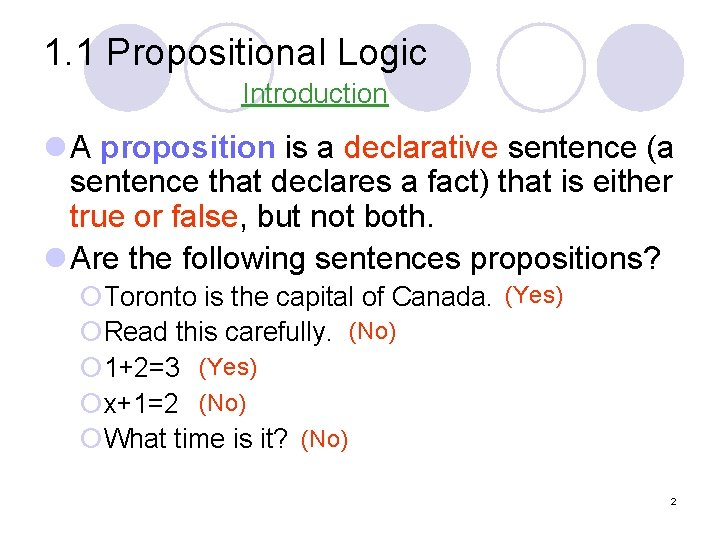1. 1 Propositional Logic Introduction l A proposition is a declarative sentence (a sentence that declares a fact) that is either true or false, but not both. l Are the following sentences propositions? ¡Toronto is the capital of Canada. (Yes) ¡Read this carefully. (No) ¡ 1+2=3 (Yes) ¡x+1=2 (No) ¡What time is it? (No) 2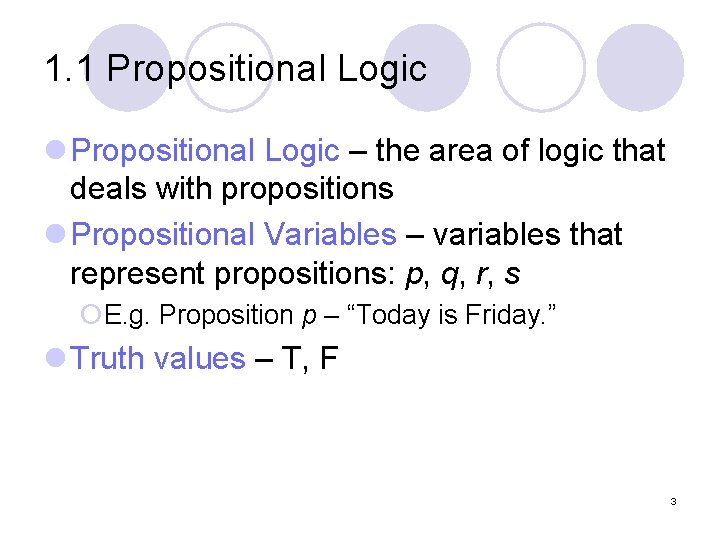1. 1 Propositional Logic l Propositional Logic – the area of logic that deals with propositions l Propositional Variables – variables that represent propositions: p, q, r, s ¡E. g. Proposition p – “Today is Friday. ” l Truth values – T, F 31. 1 Propositional Logic DEFINITION 1 Let p be a proposition. The negation of p, denoted by ¬p, is the statement “It is not the case that p. ” The proposition ¬p is read “not p. ” The truth value of the negation of p, ¬p is the opposite of the truth value of p. l Examples ¡ Find the negation of the proposition “Today is Friday. ” and express this in simple English. Solution: The negation is “It is not the case that today is Friday. ” In simple English, “Today is not Friday. ” or “It is not Friday today. ” ¡ Find the negation of the proposition “At least 10 inches of rain fell today in Miami. ” and express this in simple English. Solution: The negation is “It is not the case that at least 10 inches of rain fell today in Miami. ” In simple English, “Less than 10 inches of rain fell today in Miami. ” 4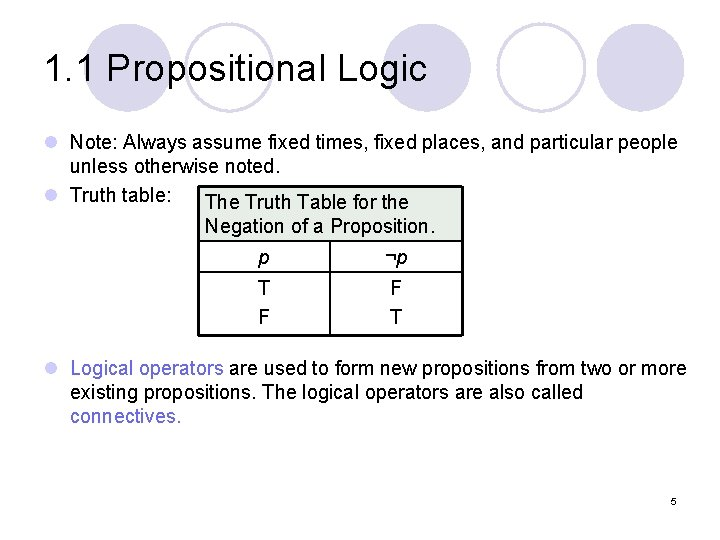1. 1 Propositional Logic l Note: Always assume fixed times, fixed places, and particular people unless otherwise noted. l Truth table: The Truth Table for the Negation of a Proposition. p ¬p T F F T l Logical operators are used to form new propositions from two or more existing propositions. The logical operators are also called connectives. 5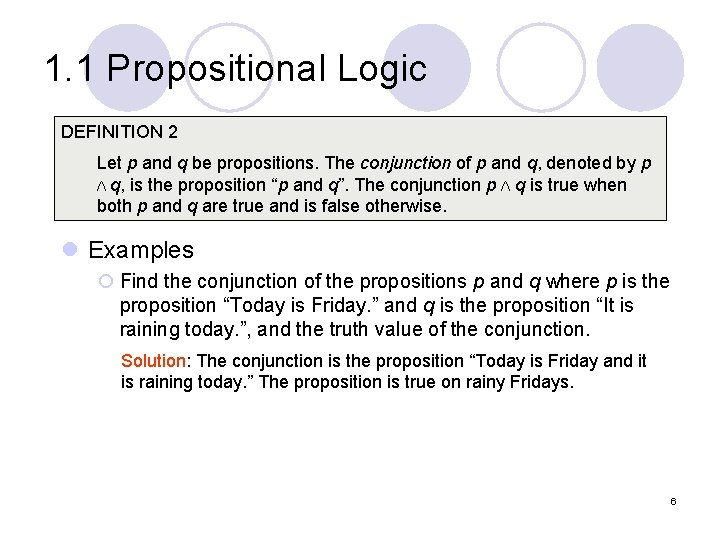1. 1 Propositional Logic DEFINITION 2 Let p and q be propositions. The conjunction of p and q, denoted by p Λ q, is the proposition “p and q”. The conjunction p Λ q is true when both p and q are true and is false otherwise. l Examples ¡ Find the conjunction of the propositions p and q where p is the proposition “Today is Friday. ” and q is the proposition “It is raining today. ”, and the truth value of the conjunction. Solution: The conjunction is the proposition “Today is Friday and it is raining today. ” The proposition is true on rainy Fridays. 61. 1 Propositional Logic DEFINITION 3 Let p and q be propositions. The disjunction of p and q, denoted by p ν q, is the proposition “p or q”. The conjunction p ν q is false when both p and q are false and is true otherwise. l Note: inclusive or : The disjunction is true when at least one of the two propositions is true. ¡ E. g. “Students who have taken calculus or computer science can take this class. ” – those who take one or both classes. exclusive or : The disjunction is true only when one of the proposition is true. ¡ E. g. “Students who have taken calculus or computer science, but not both, can take this class. ” – only those who take one of them. l Definition 3 uses inclusive or. 7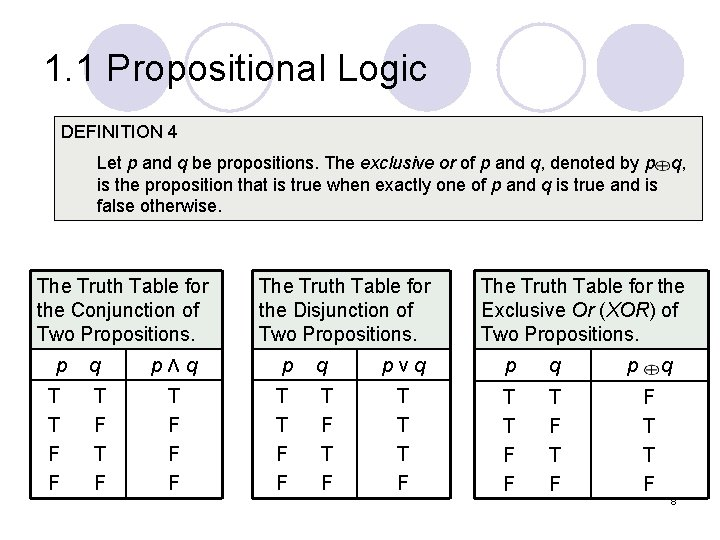1. 1 Propositional Logic DEFINITION 4 Let p and q be propositions. The exclusive or of p and q, denoted by p q, is the proposition that is true when exactly one of p and q is true and is false otherwise. The Truth Table for the Conjunction of Two Propositions. p T T F F The Truth Table for the Disjunction of Two Propositions. q pΛq p T F T F F F T T F F q T F The Truth Table for the Exclusive Or (XOR) of Two Propositions. pνq p q T T T F F T F p q F T T F 81. 1 Propositional Logic Conditional Statements DEFINITION 5 Let p and q be propositions. The conditional statement p → q, is the proposition “if p, then q. ” The conditional statement is false when p is true and q is false, and true otherwise. In the conditional statement p → q, p is called the hypothesis (or antecedent or premise) and q is called the conclusion (or consequence). l A conditional statement is also called an implication. l Example: “If I am elected, then I will lower taxes. ” p→q implication: elected, lower taxes. not elected, not lower taxes. T F F T T T F F |T |T |T |F 91. 1 Propositional Logic l Example: ¡ Let p be the statement “Maria learns discrete mathematics. ” and q the statement “Maria will find a good job. ” Express the statement p → q as a statement in English. Solution: Any of the following “If Maria learns discrete mathematics, then she will find a good job. “Maria will find a good job when she learns discrete mathematics. ” “For Maria to get a good job, it is sufficient for her to learn discrete mathematics. ” “Maria will find a good job unless she does not learn discrete mathematics. ” 10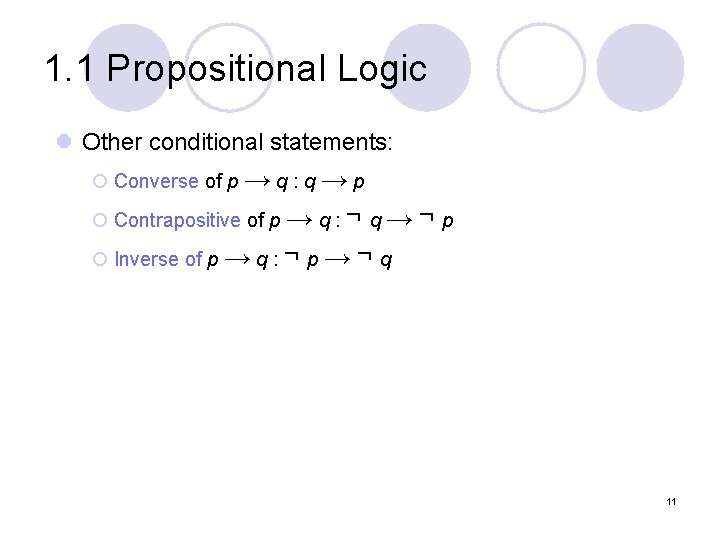1. 1 Propositional Logic l Other conditional statements: ¡ Converse of p → q : q → p ¡ Contrapositive of p → q : ¬ q → ¬ p ¡ Inverse of p → q : ¬ p → ¬ q 11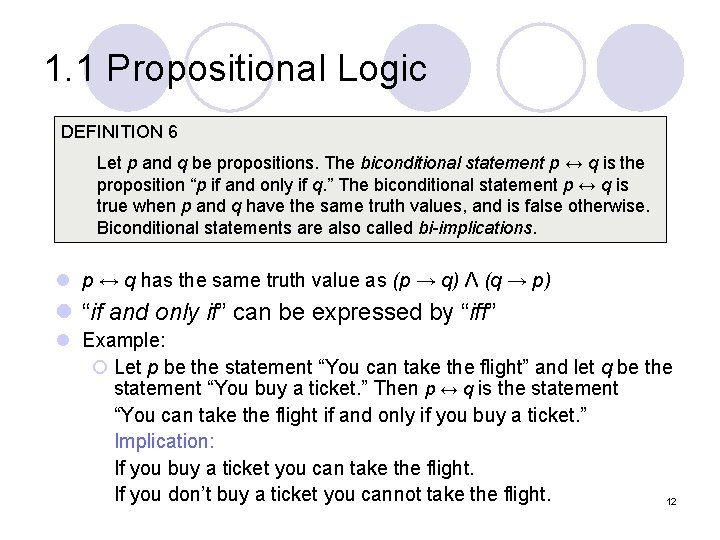1. 1 Propositional Logic DEFINITION 6 Let p and q be propositions. The biconditional statement p ↔ q is the proposition “p if and only if q. ” The biconditional statement p ↔ q is true when p and q have the same truth values, and is false otherwise. Biconditional statements are also called bi-implications. l p ↔ q has the same truth value as (p → q) Λ (q → p) l “if and only if” can be expressed by “iff” l Example: ¡ Let p be the statement “You can take the flight” and let q be the statement “You buy a ticket. ” Then p ↔ q is the statement “You can take the flight if and only if you buy a ticket. ” Implication: If you buy a ticket you can take the flight. If you don’t buy a ticket you cannot take the flight. 12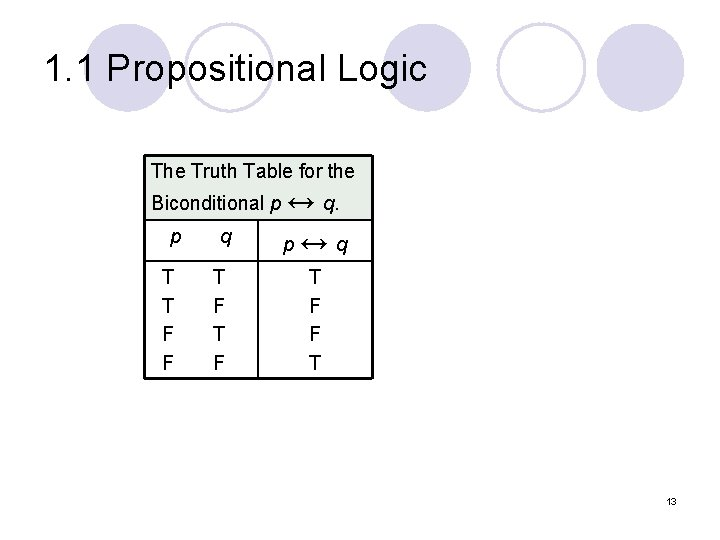1. 1 Propositional Logic The Truth Table for the Biconditional p ↔ q. p T T F F q T F p↔ q T F F T 13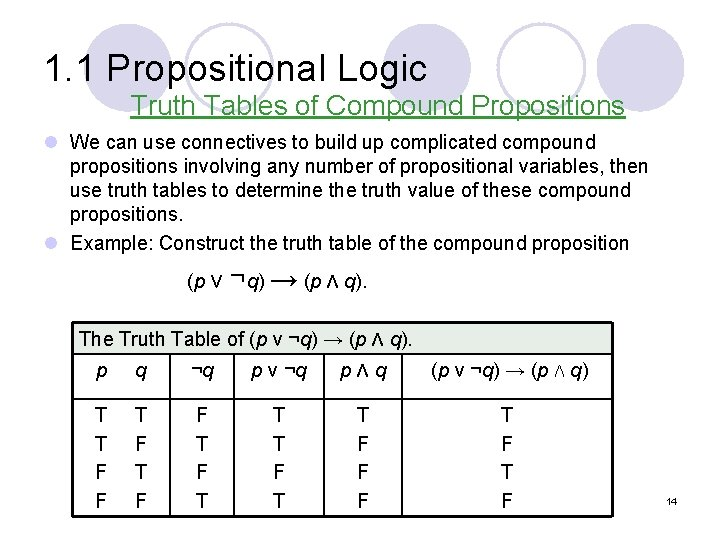1. 1 Propositional Logic Truth Tables of Compound Propositions l We can use connectives to build up complicated compound propositions involving any number of propositional variables, then use truth tables to determine the truth value of these compound propositions. l Example: Construct the truth table of the compound proposition (p ν ¬q) → (p Λ q). The Truth Table of (p ν ¬q) → (p Λ q). p q ¬q p ν ¬q pΛq (p ν ¬q) → (p Λ q) T T F F T F T T T F F F T F 14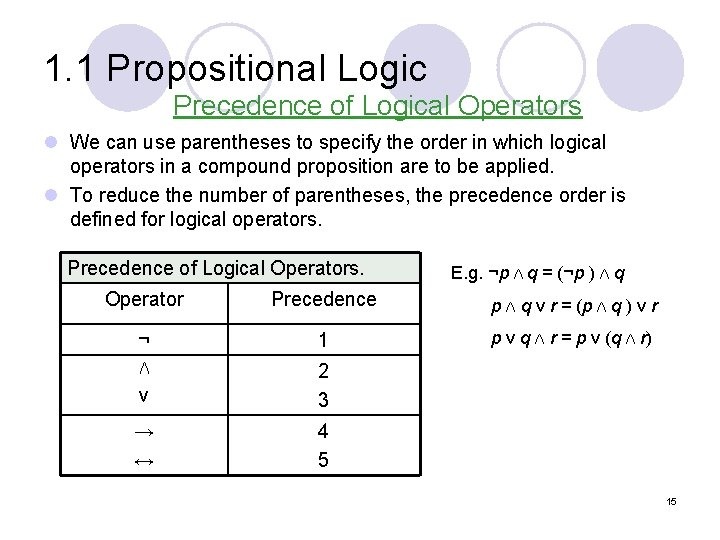1. 1 Propositional Logic Precedence of Logical Operators l We can use parentheses to specify the order in which logical operators in a compound proposition are to be applied. l To reduce the number of parentheses, the precedence order is defined for logical operators. Precedence of Logical Operators. E. g. ¬p Λ q = (¬p ) Λ q Operator Precedence p Λ q ν r = (p Λ q ) ν r ¬ 1 p ν q Λ r = p ν (q Λ r) Λ ν 2 3 → ↔ 4 5 151. 1 Propositional Logic Translating English Sentences l English (and every other human language) is often ambiguous. Translating sentences into compound statements removes the ambiguity. l Example: How can this English sentence be translated into a logical expression? “You cannot ride the roller coaster if you are under 4 feet tall unless you are older than 16 years old. ” Solution: Let q, r, and s represent “You can ride the roller coaster, ” “You are under 4 feet tall, ” and “You are older than 16 years old. ” The sentence can be translated into: (r Λ ¬ s) → ¬q. 161. 1 Propositional Logic l Example: How can this English sentence be translated into a logical expression? “You can access the Internet from campus only if you are a computer science major or you are not a freshman. ” Solution: Let a, c, and f represent “You can access the Internet from campus, ” “You are a computer science major, ” and “You are a freshman. ” The sentence can be translated into: a → (c ν ¬f). 17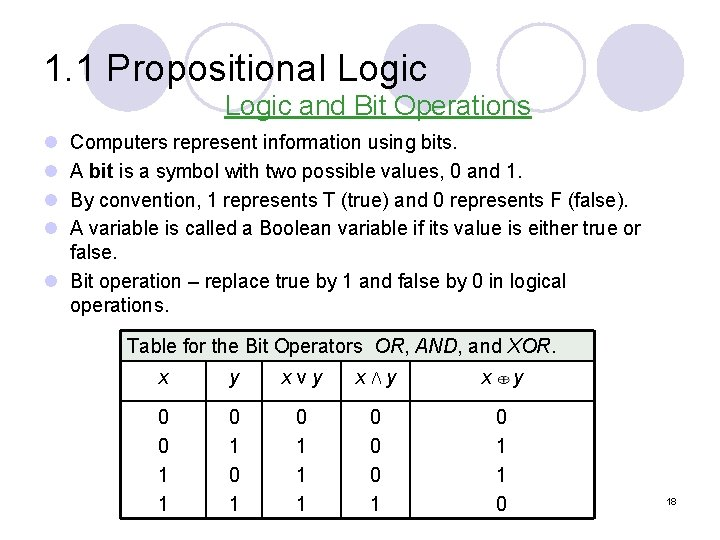1. 1 Propositional Logic and Bit Operations l l Computers represent information using bits. A bit is a symbol with two possible values, 0 and 1. By convention, 1 represents T (true) and 0 represents F (false). A variable is called a Boolean variable if its value is either true or false. l Bit operation – replace true by 1 and false by 0 in logical operations. Table for the Bit Operators OR, AND, and XOR. x y xνy xΛy 0 0 1 1 0 1 0 1 1 1 0 0 0 1 x y 0 1 1 0 181. 1 Propositional Logic DEFINITION 7 A bit string is a sequence of zero or more bits. The length of this string is the number of bits in the string. l Example: Find the bitwise OR, bitwise AND, and bitwise XOR of the bit string 01 1011 0110 and 11 0001 1101. Solution: 01 1011 0110 11 0001 1101 ---------11 1011 1111 01 0001 0100 10 1011 bitwise OR bitwise AND bitwise XOR 191. 2 Propositional Equivalences Introduction DEFINITION 1 A compound proposition that is always true, no matter what the truth values of the propositions that occurs in it, is called a tautology. A compound proposition that is always false is called a contradiction. A compound proposition that is neither a tautology or a contradiction is called a contingency. Examples of a Tautology and a Contradiction. p ¬p p ν ¬p p Λ ¬p T F F T T T F F 201. 2 Propositional Equivalences Logical Equivalences DEFINITION 2 The compound propositions p and q are called logically equivalent if p ↔ q is a tautology. The notation p ≡ q denotes that p and q are logically equivalent. l Compound propositions that have the same truth values in all possible cases are called logically equivalent. l Example: Show that ¬p ν q and p → q are logically equivalent. Truth Tables for ¬p ν q and p → q. p q ¬p ¬p ν q p→q T T F F F T T T F T T 211. 2 Propositional Equivalences l In general, 2 n rows are required if a compound proposition involves n propositional variables in order to get the combination of all truth values. l See page 24, 25 for more logical equivalences. 22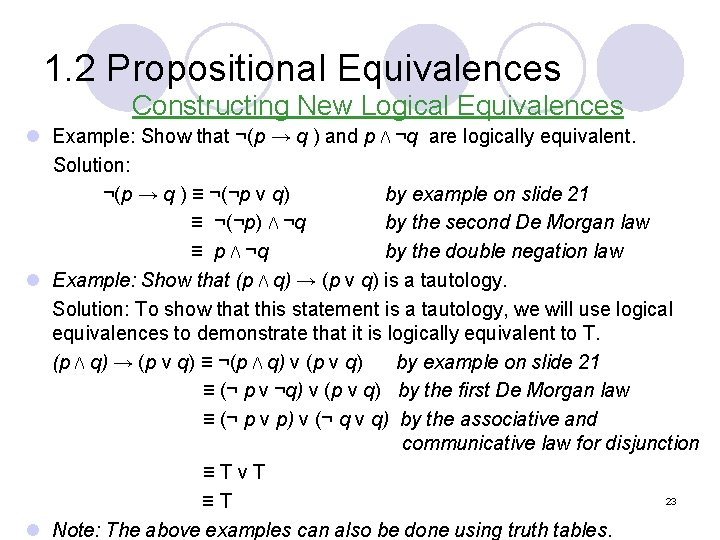1. 2 Propositional Equivalences Constructing New Logical Equivalences l Example: Show that ¬(p → q ) and p Λ ¬q are logically equivalent. Solution: ¬(p → q ) ≡ ¬(¬p ν q) by example on slide 21 ≡ ¬(¬p) Λ ¬q by the second De Morgan law ≡ p Λ ¬q by the double negation law l Example: Show that (p Λ q) → (p ν q) is a tautology. Solution: To show that this statement is a tautology, we will use logical equivalences to demonstrate that it is logically equivalent to T. (p Λ q) → (p ν q) ≡ ¬(p Λ q) ν (p ν q) by example on slide 21 ≡ (¬ p ν ¬q) ν (p ν q) by the first De Morgan law ≡ (¬ p ν p) ν (¬ q ν q) by the associative and communicative law for disjunction ≡TνT 23 ≡T l Note: The above examples can also be done using truth tables.